Get instant live expert help with Excel or Google Sheets“My Excelchat expert helped me in less than 20 minutes, saving me what would have been 5 hours of work!”

#### Post your problem and you’ll get expert help in seconds.

Your message must be at least 40 characters
Our professional experts are available now. Your privacy is guaranteed.

# How to drag a formula

In almost every worksheet in Excel, there is a formula that uses a mathematical operation or a function.  Copying formulas from one cell to another can be done through keyboard shortcuts Ctrl + C and Ctrl + V, or through copy and paste menu options.

However, Excel provides an even easier method through drag and copy.  This method can be done by the use of the fill handle.

## Fill handle

When we hover the mouse over the lower right corner of a cell or group of cells, the cursor shows a plus sign, which is the Fill Handle.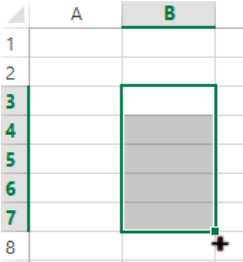Figure 1.  Fill Handle tool

The Fill Handle is a tool that enables us to copy numbers, dates or formulas in a cell down a column or across a row by simply dragging the fill handle without using any function.

## How to drag a column

It is very simple to drag a formula down a column.  We select the cell containing the formula that we want to copy, click the fill handle, hold and drag down a column or across a row.

Suppose we have a list of orders in column B and we want to calculate the cumulative sum of orders per row in column C.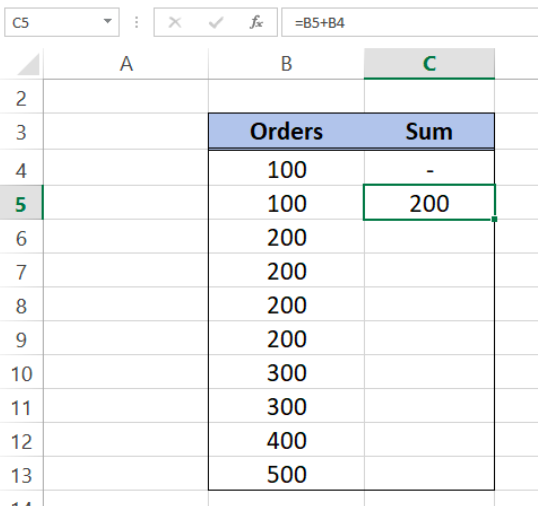Figure 2.  Sample data to drag formula down a column

The working formula in cell C5:  B5 + B4.  In order to copy the formula in C5 to cells C6 to C13, we follow these steps:

• Select cell C5
• Click the fill handle
• Hold and drag the formula down to cell C13.

With three easy steps, we are able to drag the formula and copy down the column.  We can even drag it all the way down to fill the whole column.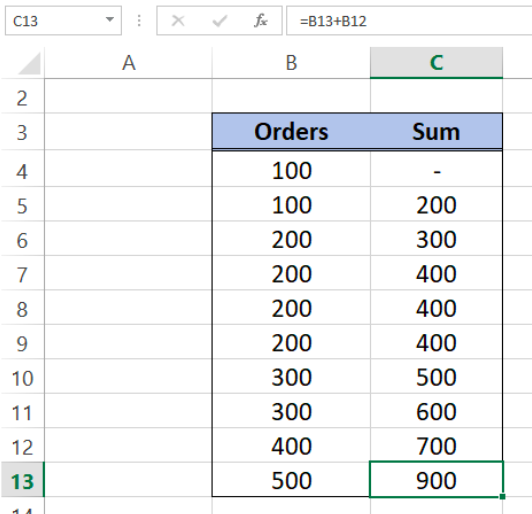Figure 3.  Output: How to drag a column

We have shown that we can easily drag a column and fill down the cells with the copied formula.  We can also drag a row and copy the formula from one cell across the cells in the same row.

## How to drag a row

Suppose we have below scores for three sets of tests.  We want to calculate the average for every set.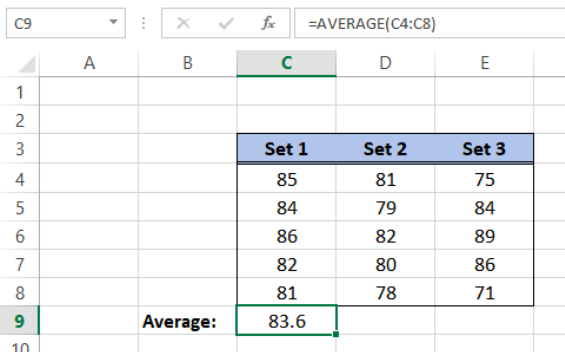Figure 4.  Sample data to drag a formula across a row

The formula for Set 1 given in cell C9 is

`=AVERAGE(C4:C8)`

In order to drag a row in Excel, we follow these steps:

• Select the cell containing the formula we want to copy, cell C9
• Click the fill handle
• Hold and drag the formula to the right up to the cell we want to fill with the Average formula, cell E9

As a result, we are able to drag and copy a formula across a row.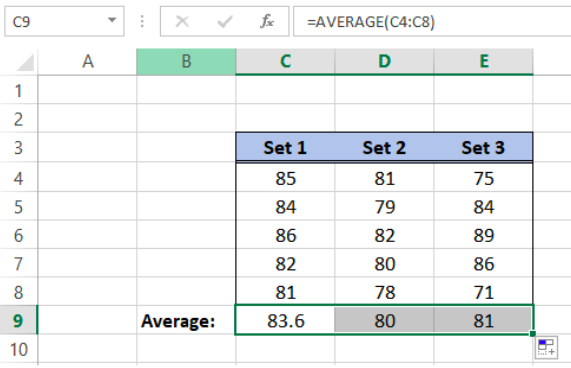Figure 5.  Output: How to drag a row

## Instant Connection to an Excel Expert

Most of the time, the problem you will need to solve will be more complex than a simple application of a formula or function. If you want to save hours of research and frustration, try our live Excelchat service! Our Excel Experts are available 24/7 to answer any Excel question you may have. We guarantee a connection within 30 seconds and a customized solution within 20 minutes.